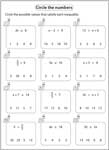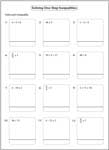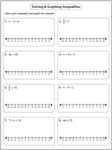One-Step Inequalities Worksheets

This page contains a huge collection of one-step inequalities worksheets based on various skills such as translating phrases, solving and graphing one-step inequalities, selecting correct graphs, identifying solutions and more. It also contains exclusive worksheets with coefficients in fractions and decimals.

Translating Phrases

Translate each verbal expression into an algebraic expression.Picking out the solutions

Circle the correct solutions that satisfy each one-step inequality.Solving One-Step Inequalities

Algebraic inequalities worksheets that takes only one step to solve each problem.Easy:

Moderate:

Difficult:

Fractions:

Decimals:

Identifying Solutions in Interval Notation

For each one-step inequality, solutions are given in the interval notations. Choose the correct solution interval for each problem.Easy:

Moderate:

Solving and Graphing One-Step Inequalities

Each worksheet has eight problems solving and graphing one-step inequalities.Easy:

Moderate:

Difficult:

Fractions:

Decimals:

Select the Correct Graph

For each one-step inequality, choose the correct graph that best describes the solution.Easy:

Moderate:

Identifying One-step Inequalities

For each solution graph, identify the correct one-step inequality.Easy:

Moderate:

Stock up your library with the complete set of worksheets on one-step inequalities.

Related Worksheets